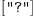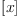login  home  contents  what's new  discussion  bug reports help  links  subscribe  changes  refresh  edit

Fortunately, FriCAS is rather easy to debug. Most of the source is very transparent, so once you have localized the bug there are mostly design issues to overcome. However, to find out which function is bugged, there are some helpful tools. These consist of:

• using Lisp debugger to localize failing function
• inspecting signatures of operations and packages, domains and categories using:
       )display operation foo
)show mydomain

• using HyperDoc to find where function is implemented: when parameters of a domain are fully specified, then clisking at "Operations" and then "Implementations" will show where each function is implemented.
• seeing which functions are called using )set message bottom on
• using the trace facility
• using profiler to identify functions that are frequently executed
• the  )set messages autoload on system command turn on information on loading files. It allows to identify most domains involved in given computation. There is a set of freqently used domains which is preloaded and there will be no messages about them

The easiest to localize are bugs which cause Lisp or algebra errors. Doing )set break break and then typing offending command should place us at Lisp debugger prompt. Then one can use backtrace command (in sbcl, when using other Lisp the command to get backtrace may be different), which will print active function invocations. Lisp debugger shows Lisp names of functions. Lisp names consist of FriCAS domain name followed by FriCAS function name inside domain possibly followed by extra info used to distinguish overloaded functions (various parts of name are separated by semicolons). For example EFSTRUC;realElem is (internal) realElem function in EFSTRUC domain, while EFSTRUC;normalize;FSF;47 is one of overloaded normalize functions.

Hint: Frequently only few top entries are interesting, in such case backtrace 6 (to get 6 top entries) may be useful. Also, FriCAS types are quite large and normally type of curent domain appear in Lisp parameter list, so FriCAS types tend to clutter display. Using (setf *print-array* nil) before backtrace command will shorten the result considerably.

Hint: To identify overloads it may be useful to look at intermediate Lisp file in .NRLIB directory of given domain. Lisp name can be used to find Lisp code and from this code correct overload can be inferred (for example looking at parameter and variable names).

Let's use the following might-be-bug as a running example:

fricas
variables((x^2+2*x)::UP(x, INT))(1)
Type: List(SingletonAsOrderedSet?)

Certainly, this is not what one would expect. So, let's find out more about variables!

One way to find all files where variables is instantiated is grep "variables.*==" *. It is not totally reliable, but I found it quite useful and it is quickly typed. Another possibility, within FriCAS, is to use

fricas
)di op variables
There are 5 exposed functions called variables :
 List(D) -> List(Symbol) from D if D has FS(D3) and D3 has COMPAR
 D -> List(Symbol) from D if D has FS(D2) and D2 has COMPAR
 D -> List(D4) from D
if D has PSCAT(D2,D3,D4) and D2 has RING and D3 has OAMON
and D4 has ORDSET
 D -> List(D4) from D
if D has PSETCAT(D2,D3,D4,D5) and D2 has RING and D3 has
OAMONS and D4 has ORDSET and D5 has RPOLCAT(D2,D3,D4)
 Fraction(Polynomial(D3)) -> List(Symbol) from RationalFunction(
D3)
if D3 has INTDOM
There are 7 unexposed functions called variables :
 SparseUnivariatePolynomial(D6) -> List(D4)
from FactoringUtilities(D3,D4,D5,D6)
if D6 has POLYCAT(D5,D3,D4) and D3 has OAMONS and D4 has
ORDSET and D5 has RING
 FortranExpression(D2,D3,D4) -> List(Symbol)
from FortranExpression(D2,D3,D4)
if D2: LIST(SYMBOL) and D3: LIST(SYMBOL) and D4 has FMTC
 D -> List(D4) from D
if D has GPOLCAT(D2,D3,D4) and D2 has Join(SRNG,ABELMON)
and D3 has OAMONS and D4 has ORDSET
 (NonNegativeInteger,PositiveInteger) -> List(D) from D if D has
JBC
 NonNegativeInteger -> List(D) from D if D has JBC
 Pattern(D2) -> List(Pattern(D2)) from Pattern(D2) if D2 has
SETCAT
 D2 -> List(D4) from PolynomialCategoryQuotientFunctions(D3,D4,D5
,D6,D2)
if D3 has OAMONS and D4 has ORDSET and D5 has RING and D6
has POLYCAT(D5,D3,D4) and D2 has FIELD with
coerce : D6 -> %
numer : % -> D6
denom : % -> D6

This is a list of all [exposed]? and [unexposed]? operations called variables, together with their [modelines]?. Note that the information that )di op (and all the other tools, like asq) give about sourcefiles is currently wrong! I use grep ")abb.* UP " * to find the correct sourcefile.

In some cases the list of operations is very long. For example, )di op coerce lists 168 exposed and 50 unexposed items. Fortunately there is another tool:

fricas
)set message bottom on
variables(x::UP(x, INT))
Function Selection for variables
Arguments: UP(x,INT)
  signature:   UP(x,INT) -> LIST(SAOS)
implemented: slot (List (SingletonAsOrderedSet))$from UP(x,INT)(2) Type: List(SingletonAsOrderedSet?) Now we know that variables does not come from some package, but rather from UP itself. You might want to compare with fricas )set message bottom on variables(x::FRAC POLY INT) Function Selection for variables Arguments: FRAC(POLY(INT)) -> no appropriate variables found in Fraction(Polynomial(Integer)) -> no appropriate variables found in Fraction(Polynomial(Integer)) Modemaps from Associated Packages no modemaps Remaining General Modemaps  Fraction(Polynomial(D3)) -> List(Symbol) from RationalFunction( D3) if D3 has INTDOM  D -> List(D4) from D if D has PSETCAT(D2,D3,D4,D5) and D2 has RING and D3 has OAMONS and D4 has ORDSET and D5 has RPOLCAT(D2,D3,D4)  D -> List(D4) from D if D has PSCAT(D2,D3,D4) and D2 has RING and D3 has OAMON and D4 has ORDSET  D -> List(Symbol) from D if D has FS(D2) and D2 has COMPAR  List(D) -> List(Symbol) from D if D has FS(D3) and D3 has COMPAR  signature: FRAC(POLY(INT)) -> LIST(SYMBOL) implemented: slot (List (Symbol))(Fraction (Polynomial (Integer))) from RF(INT)  signature: EXPR(INT) -> LIST(SYMBOL) implemented: slot (List (Symbol))$ from EXPR(INT)(3)
Type: List(Symbol)

Here, variables comes from RF. Another way to watch FriCAS looking for functions is )lisp (setq |\$monitorNewWorld| t), but I don't understand its output too well. More possibilities are discussed in the file DeveloperNotes.dvi in axiom/mnt/linux/doc/.

Given that variables comes from UP, we still don't know where it is actually coded. In fact, there is no code for variables in UP. So, where does FriCAS look next? We can find out about this using

asq -cc UP

which tells us, that the constructorcategory of UP is UPOLYC. Et voilà:

    )abbrev category UPOLYC UnivariatePolynomialCategory
...
UnivariatePolynomialCategory(R:Ring): Category ==
...
variables(p) ==
zero? p or zero?(degree p) => []
[create()]


It also helps to look at the modeline of variables, which we can find out using ')sh UP':

    )sh UP
UnivariatePolynomial(x: Symbol,R: Ring)  is a domain constructor
Abbreviation for UnivariatePolynomial is UP
This constructor is exposed in this frame.
Issue )edit /home/rubey/axiom/mnt/linux/../../src/algebra/UP.spad to see algebra source code for UP

------------------------------- Operations --------------------------------
...
variables : % -> List SingletonAsOrderedSet
...


SingletonAsOrderedSet? is a domain which consists of the single element "?"! Hence, we have reduced the problem of fixing the bug to a design issue.

 Subject:   Be Bold !! ( 14 subscribers )